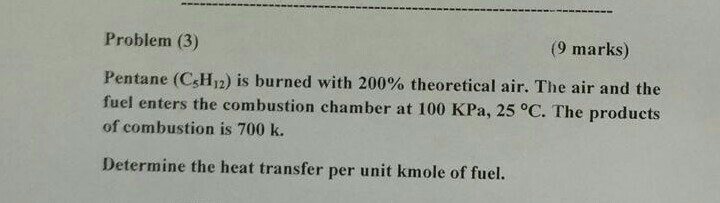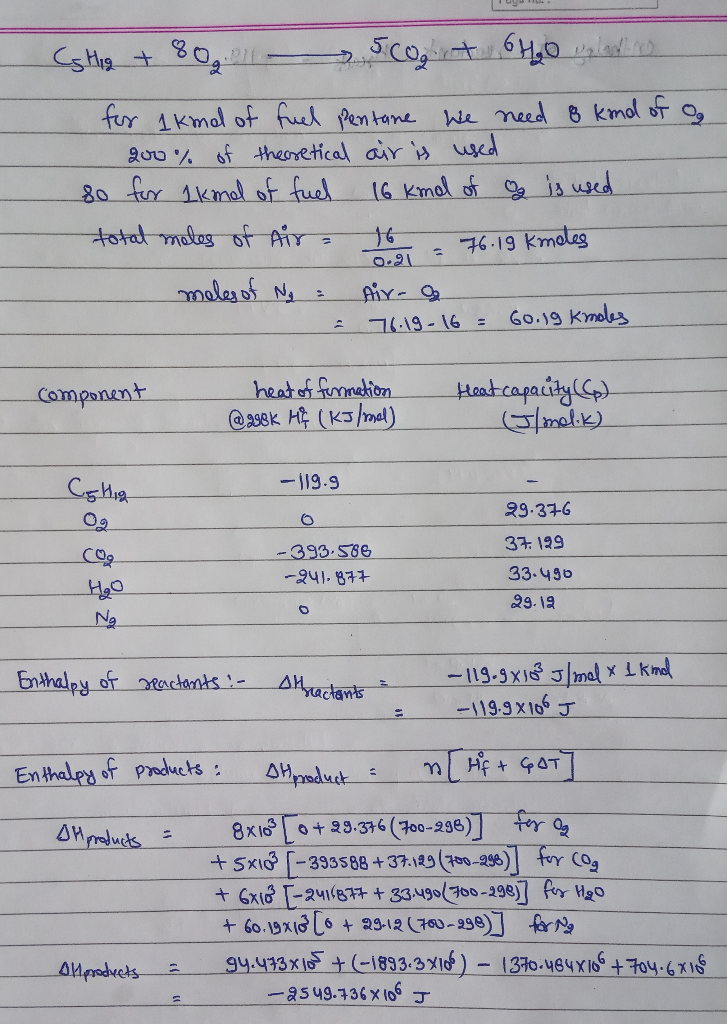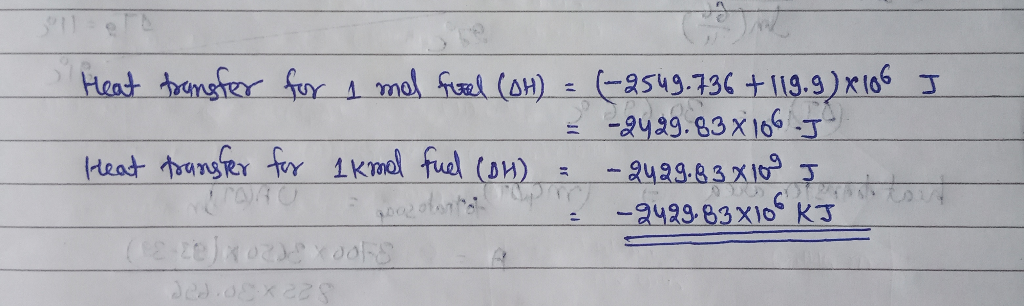Homework Help Question & Answers

(9 marks) Problem (3) Pentane (C5H12) is burned with 200% theoretical air. The air and the fuel e...(9 marks) Problem (3) Pentane (C5H12) is burned with 200% theoretical air. The air and the fuel enters the combustion chamber at 100 KPa, 25 °C. The products of combustion is 700 k. Determine the heat transfer per unit kmole of fuel.Add Answer of: (9 marks) Problem (3) Pentane (C5H12) is burned with 200% theoretical air. The air and the fuel e...
More Homework Help Questions Additional questions in this topic.

• Is the molar enthalpy of combustion of pentane the same as the molar enthalpy of formation of pentane

Need Online Homework Help?

Get FREE EXPERT Answers
WITHIN MINUTES
Related Questions